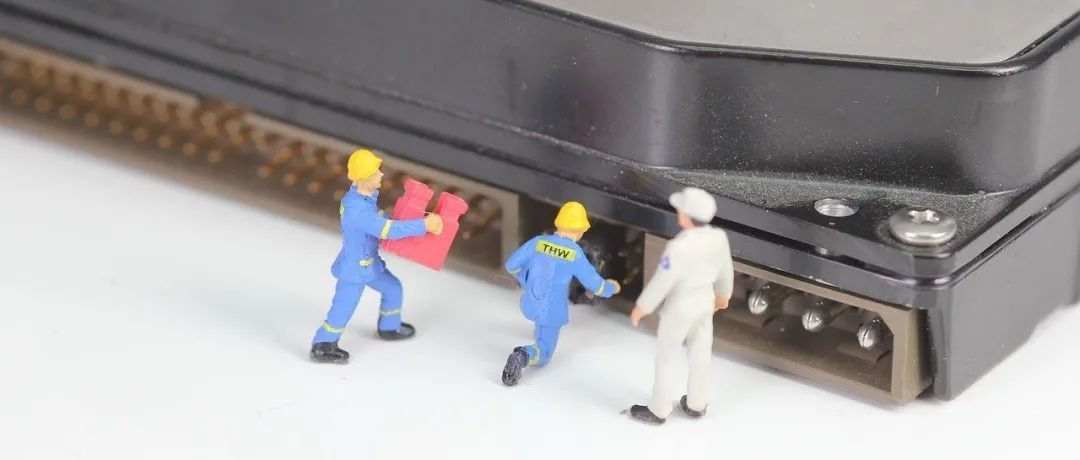## SQL数据清理及准备 – 看这一篇就够了

Excel小能手们，如何让自己数据分析及汇报的能力再上一层楼？

• 检查重复数据并将其删除
• 删除多余的空格
• 在需要时分离或组合单元格中的值
• 检查某些列中的值是否在适当的范围内
• 检查异常值
• 纠正拼写或输入错误的数据
• 向数据中添加新的相关行
• 如果缺少初始条件，则检查空值并根据其他条件检索记录。
• 检查值是否遵循准确的模式。

``````SELECT transaction_id, COUNT(transaction_id)
FROM Transaction__Table
GROUP BY transaction_id
HAVING COUNT(transaction_id) > 1;``````

``````SELECT *,
ROW_NUMBER() OVER(PARTITION BY transaction_id
ORDER BY transaction_id) AS Duplicate_row
FROM Transaction__Table
WITH CTE AS
(SELECT *,
ROW_NUMBER() OVER(PARTITION BY transaction_id
ORDER BY transaction_id) AS Duplicate_rows
FROM Transaction__Table)
DELETE FROM CTE WHERE Duplicate_rows >1;``````

``````ALTER TABLE Transaction__Table
ALTER COLUMN list_price float``````

``````SELECT REPLACE(‘So lex’, ‘ ‘, ‘’)
Then I ran a nested query to Update the brand column:
UPDATE Transaction__Table
SET brand = (SELECT REPLACE(‘So lex’, ‘ ‘, ‘’))
WHERE brand = ‘So lex’;``````

``````SELECT *
FROM Transaction__Table
WHERE LEN(transaction_id) <> 4;``````

``````UPDATE Transaction__Table
SET transaction_id = 7405
WHERE transaction_id = 7405675;``````

``````SELECT *
FROM Transaction__Table
WHERE transaction_id NOT LIKE ‘7%’;``````

``````SELECT *
FROM Transaction__Table
WHERE transaction_id IS NULL;``````

transaction_id列中没有空值，继续检查customer_id列中的空值。

``````SELECT first_name, last_name
FROM Transaction__Table
GROUP BY first_name, last_name
HAVING COUNT(*)>1;``````